求一条EXCEL的多列对比取数据的公式

t_kong 发布于 2013/05/24 21:16

年薪达不到25W大数据工程师、拿不到Offer全额退款->>>Sheet1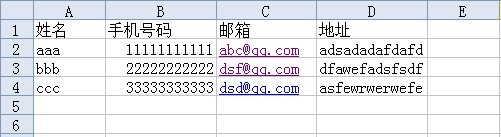Sheet2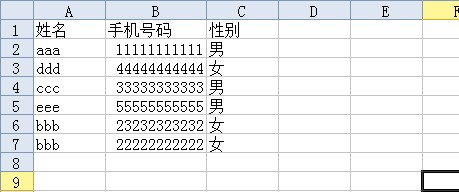0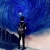Sub aa()
Dim sheet1str1, sheet1str2, sheet1str3, sheet1str4, sheet2str1, sheet2str2 As String
For i = 2 To 6 'Sheet2的总行数
sheet2str1 = Trim(Worksheets("Sheet2").Range("A" & i).Value)
sheet2str2 = Trim(Worksheets("Sheet2").Range("B" & i).Value)
For t = 2 To 4 'Sheet1的总行数
sheet1str1 = Trim(Worksheets("Sheet1").Range("A" & t).Value)
sheet1str2 = Trim(Worksheets("Sheet1").Range("B" & t).Value)
sheet1str3 = Trim(Worksheets("Sheet1").Range("C" & t).Value)
sheet1str4 = Trim(Worksheets("Sheet1").Range("D" & t).Value)
If (sheet2str1 = sheet1str1) And (sheet2str2 = sheet1str2) Then
Worksheets("Sheet2").Range("D" & i).Value = sheet1str3
Worksheets("Sheet2").Range("E" & i).Value = sheet1str4
End If
Next t
Next i
End Sub

0
t

引用来自“0day”的答案

Sub aa()
Dim sheet1str1, sheet1str2, sheet1str3, sheet1str4, sheet2str1, sheet2str2 As String
For i = 2 To 6 'Sheet2的总行数
sheet2str1 = Trim(Worksheets("Sheet2").Range("A" & i).Value)
sheet2str2 = Trim(Worksheets("Sheet2").Range("B" & i).Value)
For t = 2 To 4 'Sheet1的总行数
sheet1str1 = Trim(Worksheets("Sheet1").Range("A" & t).Value)
sheet1str2 = Trim(Worksheets("Sheet1").Range("B" & t).Value)
sheet1str3 = Trim(Worksheets("Sheet1").Range("C" & t).Value)
sheet1str4 = Trim(Worksheets("Sheet1").Range("D" & t).Value)
If (sheet2str1 = sheet1str1) And (sheet2str2 = sheet1str2) Then
Worksheets("Sheet2").Range("D" & i).Value = sheet1str3
Worksheets("Sheet2").Range("E" & i).Value = sheet1str4
End If
Next t
Next i
End Sub

0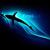Dim Dic As Object, Arr, k%, Str\$

Set Dic = CreateObject("Scripting.Dictionary")
Arr = Sheets(1).[A1].CurrentRegion
For k = 2 To UBound(Arr)
Dic(Arr(k, 1) & Arr(k, 2)) = Array(Arr(k, 3), Arr(k, 4))
Next

Arr = Sheets(2).Range("A2", [A1].End(4).Resize(1, 5))
For k = 1 To UBound(Arr)
Str = Arr(k, 1) & Arr(k, 2)
If Dic.exists(Str) Then _
Arr(k, 4) = Dic(Str)(0): Arr(k, 5) = Dic(Str)(1)
Next
Sheets(2).[A2].Resize(k - 1, 5) = Arr
Set Dic = Nothing
End Sub
0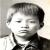引用来自“0day”的答案

Sub aa()
Dim sheet1str1, sheet1str2, sheet1str3, sheet1str4, sheet2str1, sheet2str2 As String
For i = 2 To 6 'Sheet2的总行数
sheet2str1 = Trim(Worksheets("Sheet2").Range("A" & i).Value)
sheet2str2 = Trim(Worksheets("Sheet2").Range("B" & i).Value)
For t = 2 To 4 'Sheet1的总行数
sheet1str1 = Trim(Worksheets("Sheet1").Range("A" & t).Value)
sheet1str2 = Trim(Worksheets("Sheet1").Range("B" & t).Value)
sheet1str3 = Trim(Worksheets("Sheet1").Range("C" & t).Value)
sheet1str4 = Trim(Worksheets("Sheet1").Range("D" & t).Value)
If (sheet2str1 = sheet1str1) And (sheet2str2 = sheet1str2) Then
Worksheets("Sheet2").Range("D" & i).Value = sheet1str3
Worksheets("Sheet2").Range("E" & i).Value = sheet1str4
End If
Next t
Next i
End Sub

t